Search by Topic

Resources tagged with Comparing and Ordering numbers similar to Dicey Operations in Line for Two:

Filter by: Content type:
Age range:
Challenge level:

There are 46 results

Broad Topics > Numbers and the Number System > Comparing and Ordering numbers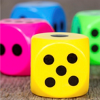Dicey Operations in Line for Two

Age 7 to 11 Challenge Level:

Dicey Operations for an adult and child. Can you get close to 1000 than your partner?Nice or Nasty for Two

Age 7 to 14 Challenge Level:

Some Games That May Be Nice or Nasty for an adult and child. Use your knowledge of place value to beat your opponent.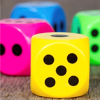Dicey Operations

Age 11 to 14 Challenge Level:

Who said that adding, subtracting, multiplying and dividing couldn't be fun?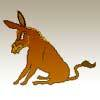Up and Down Donkey

Age 5 to 11 Challenge Level:

A number card game for 2-6 players.Nice or Nasty

Age 7 to 14 Challenge Level:

There are nasty versions of this dice game but we'll start with the nice ones...Being Curious - Primary Number

Age 5 to 11 Challenge Level:

Number problems for inquiring primary learners.The Magic Number and the Hepta-tree

Age 7 to 11 Challenge Level:

Find the exact difference between the largest ball and the smallest ball on the Hepta Tree and then use this to work out the MAGIC NUMBER!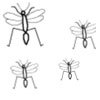Would You Rather?

Age 7 to 11 Challenge Level:

Would you rather: Have 10% of £5 or 75% of 80p? Be given 60% of 2 pizzas or 26% of 5 pizzas?Carroll Diagrams

Age 5 to 11 Challenge Level:

Use the interactivities to fill in these Carroll diagrams. How do you know where to place the numbers?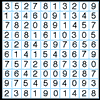A Numbered Route

Age 7 to 11 Challenge Level:

Can you draw a continuous line through 16 numbers on this grid so that the total of the numbers you pass through is as high as possible?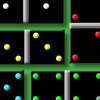Dominoes Environment

Age 5 to 11 Challenge Level:

These interactive dominoes can be dragged around the screen.Being Resourceful - Primary Number

Age 5 to 11 Challenge Level:

Number problems at primary level that require careful consideration.Multiplication Square Jigsaw

Age 7 to 11 Challenge Level:

Can you complete this jigsaw of the multiplication square?Venn Diagrams

Age 5 to 11 Challenge Level:

Use the interactivities to complete these Venn diagrams.The Thousands Game

Age 7 to 11 Challenge Level:

Each child in Class 3 took four numbers out of the bag. Who had made the highest even number?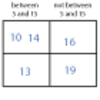More Carroll Diagrams

Age 7 to 11 Challenge Level:

How have the numbers been placed in this Carroll diagram? Which labels would you put on each row and column?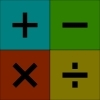Highest and Lowest

Age 7 to 11 Challenge Level:

Put operations signs between the numbers 3 4 5 6 to make the highest possible number and lowest possible number.Mathland Election

Age 11 to 14 Challenge Level:

A political commentator summed up an election result. Given that there were just four candidates and that the figures quoted were exact find the number of votes polled for each candidate.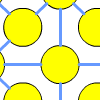One to Fifteen

Age 7 to 11 Challenge Level:

Can you put the numbers from 1 to 15 on the circles so that no consecutive numbers lie anywhere along a continuous straight line?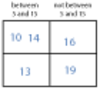More Carroll Diagrams

Age 7 to 11 Challenge Level:

How have the numbers been placed in this Carroll diagram? Which labels would you put on each row and column?Diagonal Sums

Age 7 to 11 Challenge Level:

In this 100 square, look at the green square which contains the numbers 2, 3, 12 and 13. What is the sum of the numbers that are diagonally opposite each other? What do you notice?Sort Them Out (2)

Age 7 to 11 Challenge Level:

Can you each work out the number on your card? What do you notice? How could you sort the cards?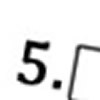Greater Than or Less Than?

Age 7 to 11 Challenge Level:

Use the numbers and symbols to make this number sentence correct. How many different ways can you find?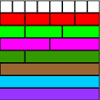Age 7 to 11 Challenge Level:

Use the fraction wall to compare the size of these fractions - you'll be amazed how it helps!Worms

Age 7 to 11 Challenge Level:

Place this "worm" on the 100 square and find the total of the four squares it covers. Keeping its head in the same place, what other totals can you make?Consecutive Seven

Age 11 to 14 Challenge Level:

Can you arrange these numbers into 7 subsets, each of three numbers, so that when the numbers in each are added together, they make seven consecutive numbers?Factor-multiple Chains

Age 7 to 11 Challenge Level:

Can you see how these factor-multiple chains work? Find the chain which contains the smallest possible numbers. How about the largest possible numbers?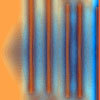Move a Match

Age 7 to 11 Challenge Level:

How can you arrange these 10 matches in four piles so that when you move one match from three of the piles into the fourth, you end up with the same arrangement?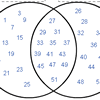Various Venns

Age 7 to 11 Challenge Level:

Use the interactivities to complete these Venn diagrams.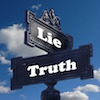Truth or Lie

Age 7 to 11 Challenge Level:

Take a look at the video of this trick. Can you perform it yourself? Why is this maths and not magic?Which Scripts?

Age 7 to 11 Challenge Level:

There are six numbers written in five different scripts. Can you sort out which is which?Even Up

Age 11 to 14 Challenge Level:

Consider all of the five digit numbers which we can form using only the digits 2, 4, 6 and 8. If these numbers are arranged in ascending order, what is the 512th number?Street Sequences

Age 5 to 11 Challenge Level:

Investigate what happens when you add house numbers along a street in different ways.Making Pathways

Age 7 to 11 Challenge Level:

Can you find different ways of creating paths using these paving slabs?Number Lines in Disguise

Age 7 to 14 Challenge Level:

Some of the numbers have fallen off Becky's number line. Can you figure out what they were?Largest Number

Age 11 to 14 Challenge Level:

What is the largest number you can make using the three digits 2, 3 and 4 in any way you like, using any operations you like? You can only use each digit once.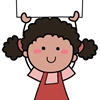Carrying Cards

Age 7 to 11 Challenge Level:

These sixteen children are standing in four lines of four, one behind the other. They are each holding a card with a number on it. Can you work out the missing numbers?Rod Fractions

Age 11 to 14 Challenge Level:

Pick two rods of different colours. Given an unlimited supply of rods of each of the two colours, how can we work out what fraction the shorter rod is of the longer one?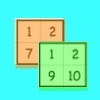Sorting the Numbers

Age 5 to 11 Challenge Level:

Complete these two jigsaws then put one on top of the other. What happens when you add the 'touching' numbers? What happens when you change the position of the jigsaws?Olympic Starters

Age 7 to 11 Challenge Level:

Look at some of the results from the Olympic Games in the past. How do you compare if you try some similar activities?Now and Then

Age 7 to 11 Challenge Level:

Look at the changes in results on some of the athletics track events at the Olympic Games in 1908 and 1948. Compare the results for 2012.Sets of Four Numbers

Age 7 to 11 Challenge Level:

There are ten children in Becky's group. Can you find a set of numbers for each of them? Are there any other sets?Euromaths

Age 11 to 14 Challenge Level:

How many ways can you write the word EUROMATHS by starting at the top left hand corner and taking the next letter by stepping one step down or one step to the right in a 5x5 array?Writ Large

Age 11 to 14 Challenge Level:

Suppose you had to begin the never ending task of writing out the natural numbers: 1, 2, 3, 4, 5.... and so on. What would be the 1000th digit you would write down.Farey Sequences

Age 11 to 14 Challenge Level:

There are lots of ideas to explore in these sequences of ordered fractions.Greetings

Age 11 to 14 Challenge Level:

From a group of any 4 students in a class of 30, each has exchanged Christmas cards with the other three. Show that some students have exchanged cards with all the other students in the class. How. . . .